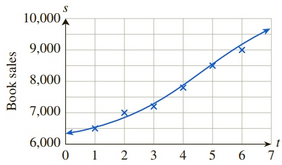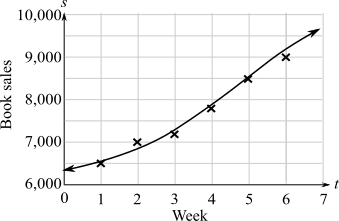Chapter 11, Problem 67REFinite Mathematics and Applied Cal...

7th Edition
Stefan Waner + 1 other
ISBN: 9781337274203

Solutions

Chapter
SectionFinite Mathematics and Applied Cal...

7th Edition
Stefan Waner + 1 other
ISBN: 9781337274203
Textbook Problem

Sales OHaganBooks.com decided that the cubic curve in Exercise 57 was not suitable for extrapolation, so instead it tried s ( t ) = 6 , 000 + 4 , 500 1 + e 0.55 ( t − 4.8 ) , as shown in the following graph:Weeka. Compute s ' ( t ) , and use the answer to estimate the rate of increase of weekly sales at the beginning of the seventh week ( t = 6 ) . (Round your answer to the nearest unit.)b. Compute lim t → + ∞ s ' ( t ) , and interpret the answer.

(a)

To determine

To calculate: The value of S(t) and determine the rate of weekly sale increased in the starting of the seventh week if the graph of the function s(t)=6000+4,5001+e0.55(t4.8) represents the weekly sale of the books on the website of OHaganBooks.com is,Explanation

Given information:

The graph of the function s(t)=6000+4,5001+e0.55(t4.8) represents the weekly sale of the books on the website of OHaganBooks.com is,

Formula used:

Constant multiple rules of derivative of a function f(x) are f'(cx)=cf'(x) where c is constant.

The derivative of a function f(x)=un using the chain rule is f(x)=ddx(un)=nun1dudx where u is the function of x.

The derivative of e raised to a function is ddxeu=eududx.

Calculation:

Consider the weekly sale of the books, s(t)=6000+4,5001+e0.55(t4.8)

To compute S(t) take the derivative of the equation,

s(t)=ddt(6000+4,5001+e0.55(t4.8))=ddt(6000)+ddt(4,5001+e0.55(t4.8))=0+ddt(4,5001+e0.55(t4.8))=ddt(4,5001+e0.55(t4.8))

Apply the constant multiple rules,

s(t)=4500ddt(11+e0.55(t4.8))

Rewrite the expression in power form as

s(t)=4500ddt(1+e0.55(t4.8))1

Now apply chain rule,

s(t)=4500(1)(1+e0.55(t4.8))2ddt(1+e0.55(t4.8))=4500(1+e0.55(t4.8))2(ddt(1)+ddt(e0.55(t4.8)))=4500(1+e0.55(t4.8))2(0+ddt(e0.55(t4.8)))=4500(1+e0.55(t4.8))2ddt(e0

(b)

To determine

To calculate: The value of limt+s(t) and interpret the answer if, the graph of the function s(t)=6000+4,5001+e0.55(t4.8) represents the weekly sale of the books on the website of OHaganBooks.com is,Still sussing out bartleby?

Check out a sample textbook solution.

See a sample solution

The Solution to Your Study Problems

Bartleby provides explanations to thousands of textbook problems written by our experts, many with advanced degrees!

Get Started

In Exercises 7-28, perform the indicated operations and simplify each expression. 14. xexx+1+ex

Applied Calculus for the Managerial, Life, and Social Sciences: A Brief Approach

Is 460 divisible by3?

Elementary Technical Mathematics

Elimination of the parameter in x = 2t3/2, y = t2/3 gives: x4 = 16y9 16x4 = y4 x3 = 8y4 8x3 = y4

Study Guide for Stewart's Single Variable Calculus: Early Transcendentals, 8th